# Inclusive Practices in Math

### Asynchronous Math for ALL Training

#### Math for ALL Asynchronous Courses through tealearn.com

Through hands-on activities, participants will explore how learning abilities associated with disabilities, including dyslexia and disorders related to dyslexia, impact the learning of whole number addition and subtraction. In this session, participants will build knowledge and understanding of how instructional supports, including the Universal Design for Learning (UDL) Guidelines along with assistive technology, can be used to accommodate and reduce the impact of a disability.

Registration is FREE!

Register for Math for ALL

 “The state of Texas provides special education-related services to approximately 500,000 students. However, only 41 percent of these students are approaching grade level knowledge and skills in reading and math compared to 75 percent of all Texas students who are approaching grade level”.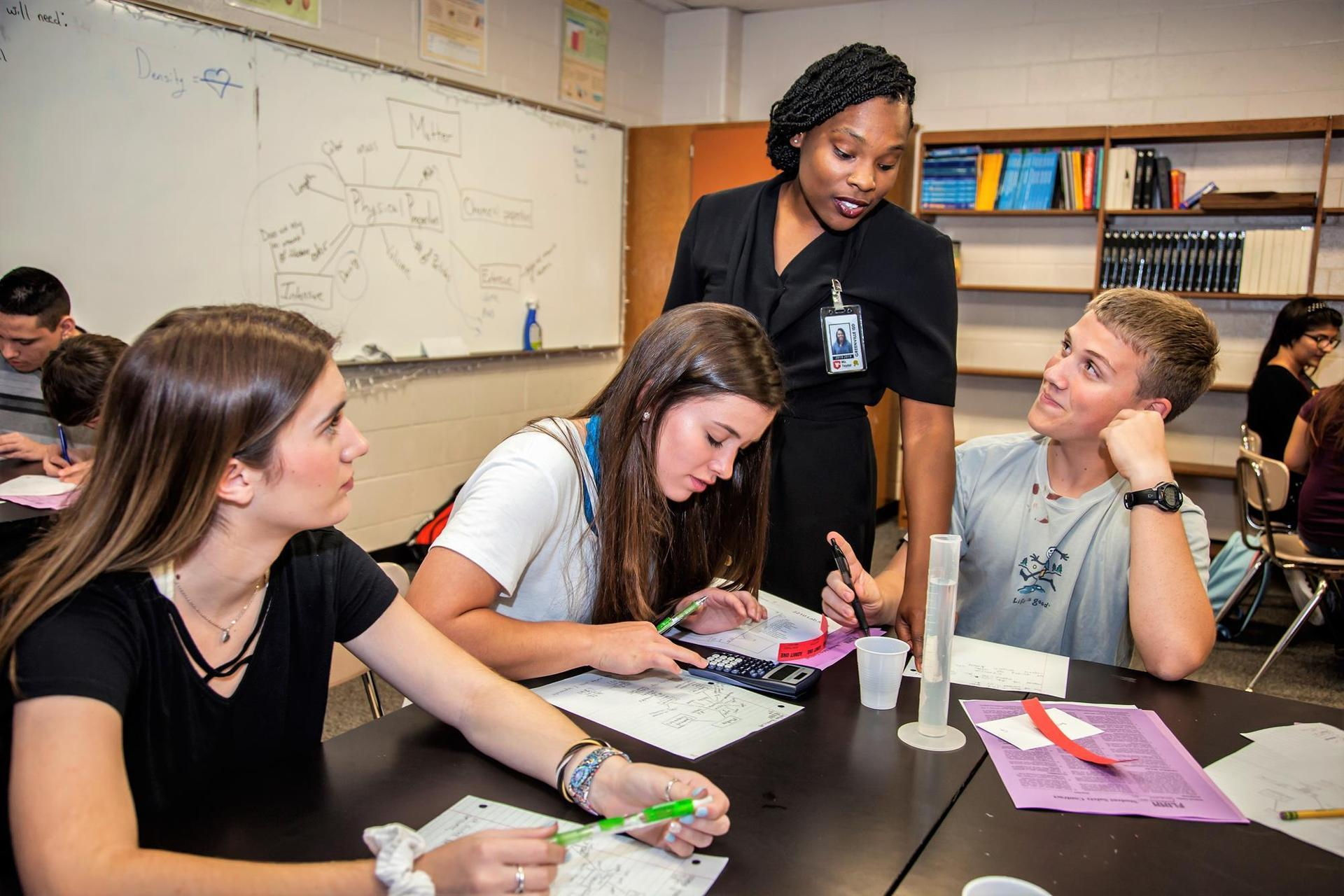(Texas Education Agency Special Education Strategies Plan, 2018).

## Understanding Math Through a Progressive Approach Model

Effectively teaching mathematics begins with the understanding that students must master the vocabulary of mathematics before grasping the more advanced principles and structures. Mary Austin (1955), explains that a student's comprehension of math includes two parts. First, students must understand how the meanings of math specific words are different from the more general meanings. Second, teachers must understand that math vocabulary is specialized and should be taught in a way that supports mathematic comprehension.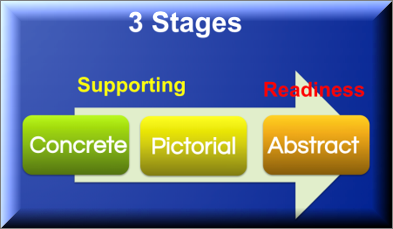The concrete, pictorial, abstract (CPA) approach was proposed by Jerome Bruner as a way of scaffolding learning. Dr. Yeap Ban Har, and others, have incorporated these three stages in the teaching of mathematics. The CPA approach is part of a vertical alignment that captures a progressive approach to instill in students the comprehensive aspect of mathematics. Teaching math using the CPA progression supports the TEKS readiness and supporting standards, which are evaluated on the STAAR assessment. For a student to understand and apply math, it is important that they are able to connect a strong foundational vocabulary to this progressive approach.

### Background KnowledgeMath is more than a set of skills to learn, it is a comprehensive way of thinking. Understanding math is not simply determining what numbers can do. True mathematical understanding requires the background knowledge of both how the numbers work together and the processes used to find solutions. Math mastery requires a strong foundation of understanding math language that includes numbers, symbols, and words. It also requires an understanding that words represent specific mathematical symbols. Students need to know that the words they write or say can be replaced with numbers or other mathematical symbols that have the same meaning as the words.

### Vocabulary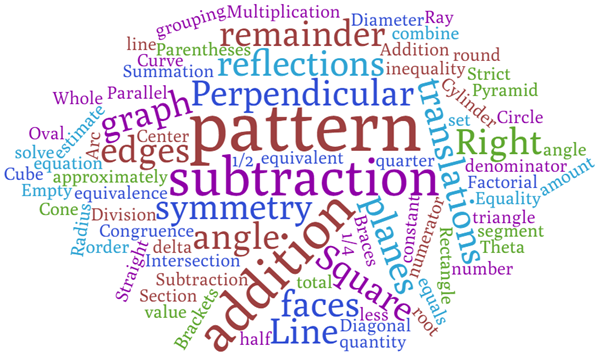"Development of vocabulary is crucial to any experience involving language. Because of the high incidence of unfamiliar vocabulary in mathematics, teaching unknown words becomes central to mathematics literacy." Monroe and Orme (2002)

Math vocabulary is presented differently than vocabulary presented in other instructional settings. In the world of math, vocabulary is not presented as formal definitions and examples. Student mastery of math vocabulary is evidenced by the ability to construct an understanding around a concept, as opposed to learning the formal definition. Because math is about a process, a student needs to be able to connect the process to math terms. Strategies used when reading, such as decoding words and using contextual evidence, can also support the learning of mathematical vocabulary.

## Math Model Vertical Alignment

The CPA approach was first developed by American psychologist, Jerome Bruner, and researched heavily under Dr. Yeap Ban Har. CPA is a technique that helps build the contextual understanding of how math works. The CPA model is utilized in all the stages of the vertical progression of math. This approach requires students to build on concrete mathematical understandings through using manipulatives or pictures to validate their thinking and understanding of math concepts. In order to progress to the abstract phase of math, the student needs a solid foundation in the concrete and pictorial phases.
The concrete phase, known as the “doing” stage, is the first step in conceptualizing a math concept. This is the foundation phase that connects concept to experience eventually leading to abstract thinking (Pashler, et al. 2007). Students need to understand how things work before they can determine how to solve problems. This stage is all about the student having a chance to learn physically using hands-on manipulatives.

Learning Target:

I will gain a better understanding of the math progression by building my capacity to use manipulatives.

Definition of Manipulatives:

Objects or tools that provide a concrete way for students to bring meaning to a mathematical idea.

How to engage the student:

Most face-to-face math classes have counters such as two-sided plastic circles, base ten blocks, counting bears, and Cuisenaire rods. However, manipulatives can be pennies, beans, buttons, gummy bears, or any other small object the student can touch and move. Let the student explore and give a value to the objects they use. Try to make sure all of the manipulatives are all the same (e.g., 5 pennies, not 3 pennies and 2 beans) when used with a specific lesson. This helps students learn that a specific object has a value.

What it looks like in a classroom:

Teachers use the appropriate manipulatives to model a math problem. Students practice using manipulatives during their independent work. Manipulatives can be used to visually represent a student’s thinking process in solving a problem.

• Addition/Subtraction – two-sided counters, counting bears, base ten blocks

• Multiplication/Division – two-sided counters, counting bears, base ten blocks, dry erase markers (to draw the circle to help identify the equal groups)

• Fractions – fraction strips, Cuisenaire rods, two-sided counters

What it looks like in action:

Using manipulatives supports instruction and helps build capacity for a student to learn how to use objects to represent a problem.

Note: In a student’s individualized education program (IEP), certain manipulatives may be allowed on state assessments. Check their IEP and TEA guidance on testing accommodations for math.
Learning Target:

I will gain a better understanding of the math progression by building capacity to connect manipulatives to a picture in a 2D format.

Definition of Pictures:

visual ways of showing a value in math

How to engage the student:

In this phase, students start applying their drawings or pictures to word problems, taking what they visualize it you might see three lines or circles, a plus sign, and two additional lines or circles. You might also see circles to group numbers. Model Drawing is another strategy and requires students to draw rectangular boxes to represent part-whole relationships and math values in a math problem. It might look similar to this.

How pictures support math learning:

Pictures help students understand how the elements in a math problem relate to each other. Drawing allows the student to simplify the problem in order to identify a problem-solving plan that can be applied to similar problems. It also helps students transform the problem into a mathematical form.

What it looks like in the classroom:

Teachers model what a problem would look like using simple drawings to represent the math values.

 Addition/Subtraction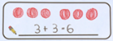Multiplication/Division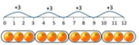Fractions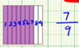What it looks like in action:

On an independent activity, students draw out their thinking or visualization of the content from a word problem. Many schools use mnemonics that help identify steps in the problem-solving plans. The student's us of pictures or visualization is usually the "action" or "explain your thinking step" in a problem-solving plan. This is a critical step for students. Similar to reading comprehension, visualization of the content leads to a deeper understanding.

Example problems assessing a student's understanding of the use of pictures to represent math values (fourth grade released 2019 test)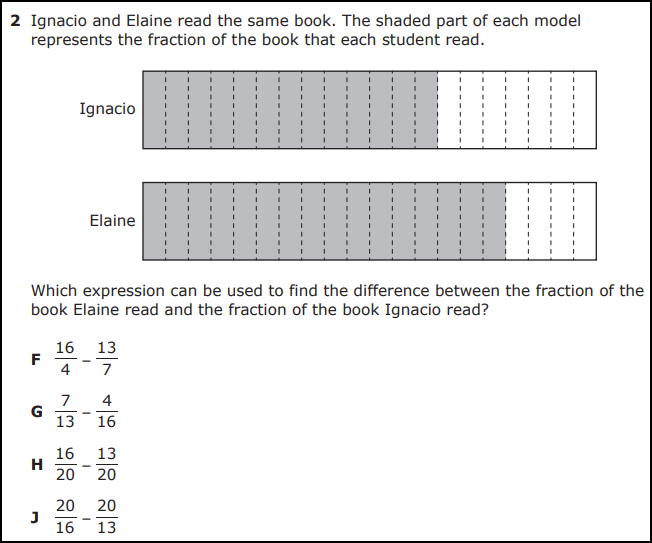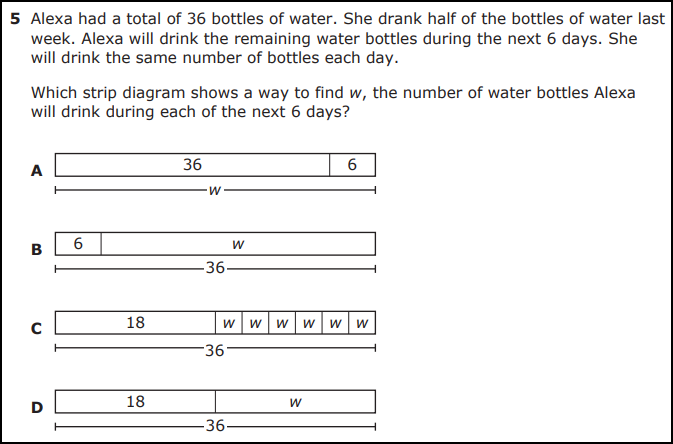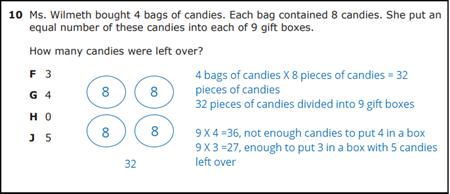Learning Target:

I will gain a better understanding of the math progression by showing the connection to abstract thinking and diving deeper into the mental process of math.

Definition of Abstract:

The process of working through a math problem using only abstract symbols such as numbers, notation, and mathematical symbols (+, -, x, /). Students do not use manipulatives or drawings.

How to engage the student:

Abstract thinking is a hard concept for many students. Most math problems on a state mandated test progress towards the abstract thinking problem. Students engage in learning when they are actively working through the problem solving process. Helping students connect the patterns to properties and mathematical structures supports developing reasoning skills.

How abstract thinking supports math learning:

Progressing through the phases of learning math concepts leads to the ability to think about abstract concepts such as algebraic reasoning.

What it looks like in the classroom:

Through teaching problem-solving strategies, teachers demonstrate how to use abstract thinking to determine what is required to solve a math problem. Some examples are, but are not limited to:

• Multiplication/Division - teaching students how to group, how to multiply to power of zeroes, and halving and doubling practices
• Fractions - teaching how to find like denominators and connecting fractions to the value of a dollar to make adding or subtracting of fractions easier

What it looks like in action:

On an independent activity, students use only abstract symbols to solve a problem.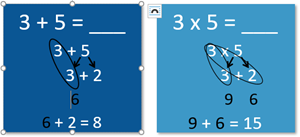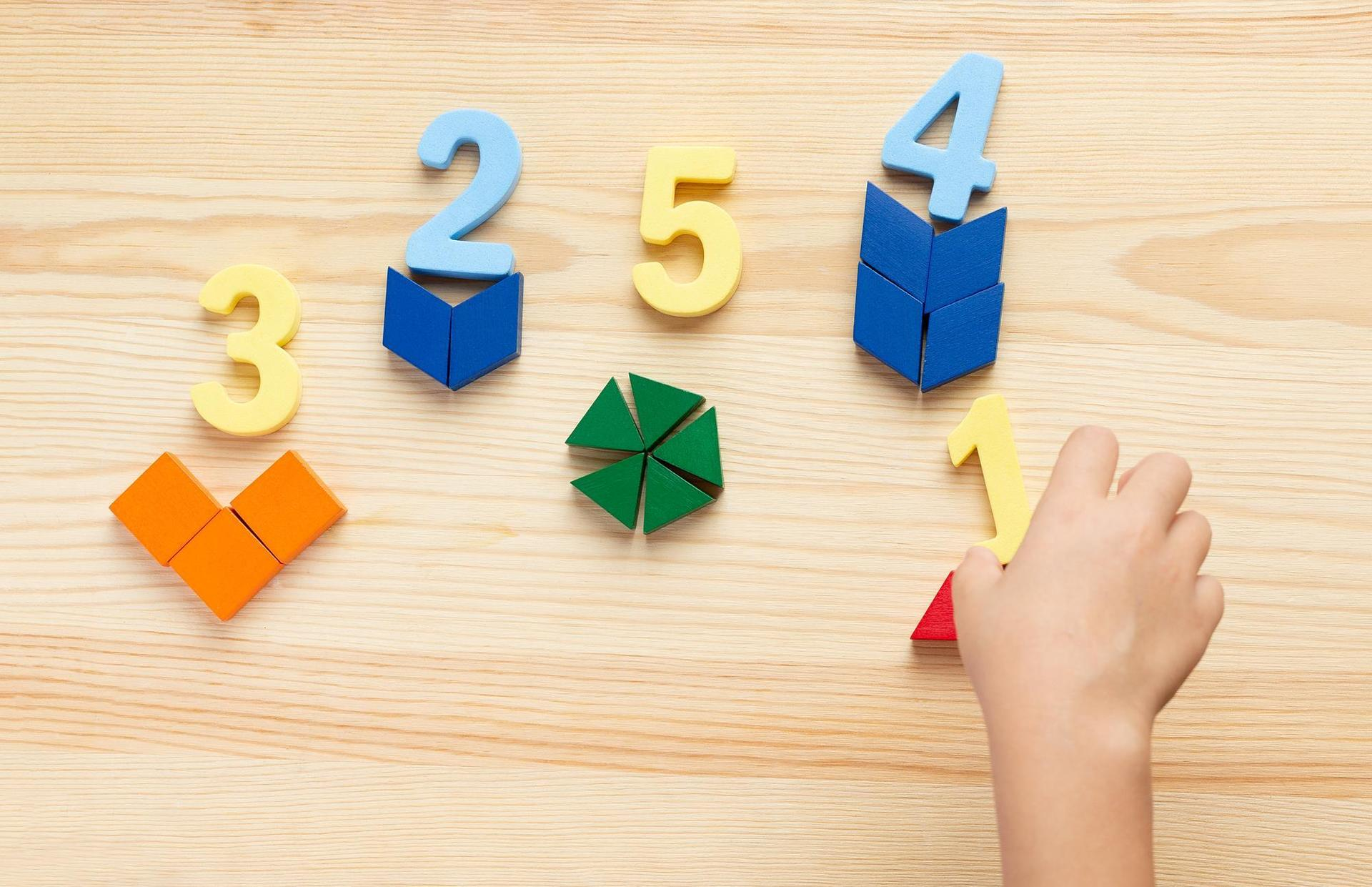The counting phase begins as students learn to recognize numbers in their surroundings. Number recognition, one to one correspondence, and subitizing (being able to recognize numbers in different forms) are part of the counting progression. Students have number recognition when they are able to recognize numbers visually. Also, in this stage, students learn to recognize patterns and sequences.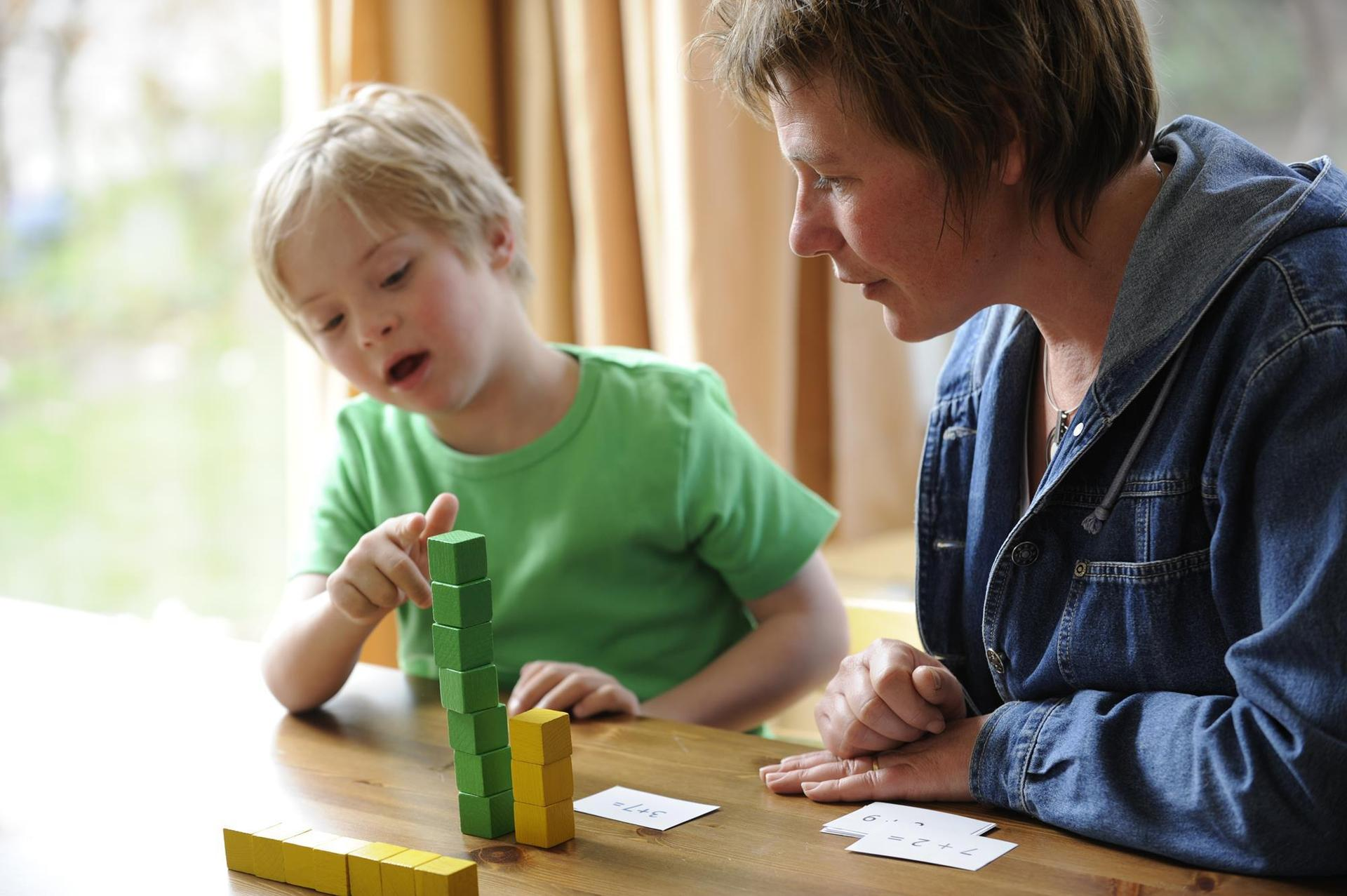Students learn how to join and combine numbers in the additive phase. They understand numbers through part-whole. Subtraction is also introduced in this phase. Subtraction is the ability to separate and reduce numbers. Students learn how to group numbers to make a sum or a difference. These progressions build upon counting and number recognition.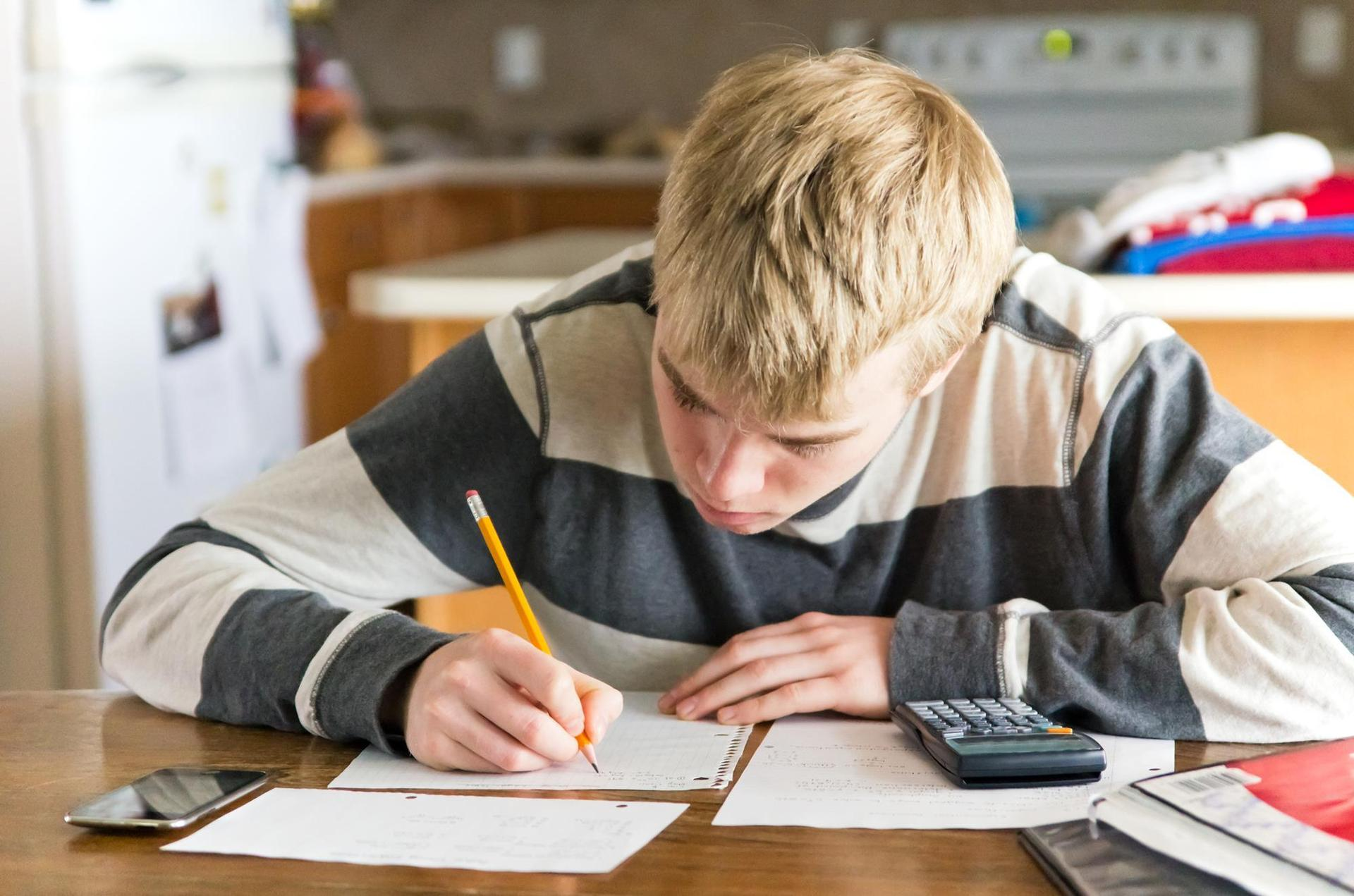In the multiplicative phase, students build their understanding that patterns and relationships are advanced additive or subtractive. Students learn how to construct numbers, which eventually leads to the ability to create equations and engage in algebraic reasoning. Multiplication is advanced repetitive addition while division is advanced repetitive subtraction.In the proportional phase, students build their understanding of relationships between numbers. Students are able to create equations. They can explain proportions and ratios, and how they relate to the multiplicative processes. When two ratios are equal, it is said to be proportional. We see proportions in fractions and ratios.Finally, algebraic reasoning is the critical extension of number sense. Students learn how to apply numbers and other mathematical understandings to problem solving. Algebraic thinking enables students to recognize and analyze patterns.

## New Resource!Instructional Routines for Mathematics Intervention

### Designed for Texas Teachers and Aligned with the TEKS

These free materials contain 23 modules that are focused on different mathematics content and are designed for grades 2 through 8.

These modules feature:
• Step-by-step routines for explicit teaching of the mathematics content.
• Vocabulary Cards with visuals that can be easily displayed in the classroom.
• Problem Sets with greater than 50 ready-to-use problems for each module.